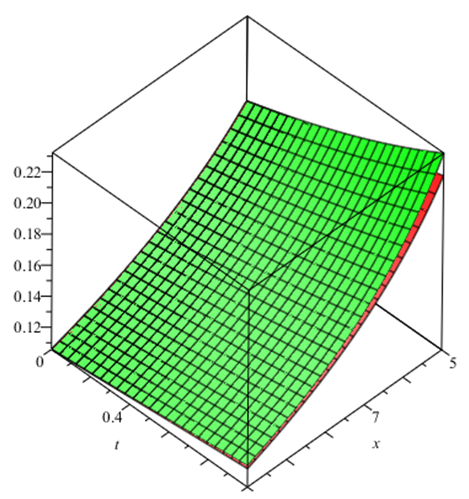American Journal of Numerical Analysis
ISSN (Print): 2372-2118 ISSN (Online): 2372-2126 Editor-in-chief: Emanuele Galligani
Journal Browser
Article Metrics
• 12202
Views
• 6998
Saves
• 2
Citations
• 38
Likes
Export Article
American Journal of Numerical Analysis. 2014, 2(1), 11-13
DOI: 10.12691/ajna-2-1-3
Open AccessArticle

### Application of VIM Method for Nonlinear Porous Media Equations

1Department of Applied Mathematics, Tonekabon Branch, Islamic Azad University, Tonekabon, Iran

2Department of Applied Mathematics, Shahid Beheshti University, Tehran, Iran

Pub. Date: January 03, 2014

Cite this paper:
E. Abdolmaleki and S.A. Yousefi. Application of VIM Method for Nonlinear Porous Media Equations. American Journal of Numerical Analysis. 2014; 2(1):11-13. doi: 10.12691/ajna-2-1-3

### Abstract

In this paper, we applied He’s variational iteration method (VIM) to solve nonlinear porous media equations. The main advantage of this method is the flexibility to give approximate solutions to nonlinear problems without linearization or discretization. The results show that this method is simple and effective.This work is licensed under a Creative Commons Attribution 4.0 International License. To view a copy of this license, visit http://creativecommons.org/licenses/by/4.0/

### FiguresFigure of 2

### References:

  S. Pamuk, Solution of the porous media equation by Adomion’s decomposition method, phys.lett. A 344 (2005) 184-188.  T. P. Witelski, J. Math. Biol. 35 (1997) 695.  A. D. Polyanin, V. F. Zaitsev, Handbook of Nonlinear Partial Differential Equations, Chapman & Hall / CRC press, Boca Raton, 2004.  J. H. He, Variational iteration method for delay differential equations, commun. Nonlinear Sci. Number. Simul. 2 (4) (1997) 235-236.  J. H. He, Approximate analytical solution for seepage flow with fractional derivatives in porous media, Comput. Methods Appl. Mech. Engry. 167 (1998) 57-68.  J. H. He, Approximate solution of nonlinear differential equation with convolution product nonlinearities, Comput. Methods Appl. Mech. Enggr. 167 (1998) 69-73.  J. H. He, Variational iteration method-a kind of nonlinear analytical technique: some examples, Int. J. Non-linear Mech. 34 (1999) 699-708.  M. Inokuti, et al., General use of the Lagrange multiplier in non-linear mathematical physics, in: S, Nemat-Nasser (Ed.), Variational Method in the Mechanics of solids, pergamon Press, Oxford. 1978, pp. 156-162.  M. Tatari, M. Dehghan, On the convergence of He’s variational iteration method, J. Comput. Appl. Math. 207 (2007) 121-128.  G.C. Wu, D. Baleanuc, Variational iteration method for the Burgers’ flow with fractional derivatives-New Lagrange multipliers, 37 (2013), 6183-6190.  A. Maidia, J.P. Corriou, Open-loop optimal controller design using variational iteration method, Applied Mathematics and Computation,219 (2013) 8632-8645.  M. Tatari. M. Dehghan, solution of problems in calclus of variations via He’s variational iteration method, phys. Lett. A362 (2007) 401-406.  M. Dehghan, F. Shakeri, Application of He’s variational iteration method for solving the Cauchy reaction diffusion problem, J. Comput. Appl. Math. 214 (2008) 435-446.  F. Shakeri, M. Dehghan, Numerical solution of the Klien-Gordon equation via He’s variational iteration Method, Nonlinear Dynam. 51 (2008) 89-97.  M. Dehghan, M. Tatari, Identifying an unknown function in a parabolic equation with over specified data via He’s variational iteration method, chaos solitons Fractals 36 (2008) 157-166.  M. Dehghan, F. shakeri, Numerical solution of a biological Population model using He’s variational iteration method, comput. Math. Appl. 54 (2007) 1197-1209.  M. Dehghan, Finite difference procedures for solving a problem arising in modeling and design of certain optoelectronic devices, Math. Comput. Simulation 71 (2006) 16-30.  M. shakourfar, M. Dehghan, on the numerical solution of nonlinear systems of volterla integro-differential equations with delay arguments, computing 82 (2008) 241-260.  M. Dehghan, M. Shakourifar, A. Hamidi, The solution of linear and nonlinear systems of Volterra functional equations using Adomian-Pade technique, Chao, solitons and fractals 39 (2009) 2509-2521.  M. Tatari, M. Dehghan, He’s variational iteration method for computing a control parameter in a semi-linear inverse parabolic equation, chaos, solitions and Fractals 33 (2007) 671-677.  M. Tatari, M. Dehghan, Improvement of He’s variational iteration method for solving systems of differential equation s, computers and Mathematics whit Applications 58 (11-12) (2009) 2160-2166.  M. Dehghan, F. Shakeri, solution of paravolic integro-differential equations arising in heat conduction in materials with memory via He’s variational iteration technique, communication in Numerical Methods in Engineering (2008) (inpress).  S. Momani, S. Abuasad, Application of He’s variational iteration method to Helmholtz equation, chao solitons Fractals 27 (5) (2006) 1119-1123.  H. Tari, D. D. Ganji, M. Rostamian, Approximate solutions of K (2, 2), kdv and modified kdv and modified kdv equations by variabional iteration method, homotopy perturbation method and homotopy analysis method, Int. J. Nonlinear Sci. Numer. Simul. 8 (2) (2007) 203-210.  S. Momani, Z. Odibat, Numerical comparison of methods for solving linear differential equations of fractional order, chao solution Fractals 31 (2007) 1238-1255.  Z. M. Odibat, S. Momani, Application of variational iteration method to Nonlinear differential equations of fractional order, Int. J. Nonlinear Sci. Numer. Simul. 7 (2006) 27-34.  N. Bildik, A. Konuralp, The use of variational iteration method, differential transform method and Adomian decomposition method for solving different types of nonlinear partial differential equations, Int. J. Nonliner Sci. Numer. Simul. 7 (2006) 65-70.  J. H. He, X.H. Wu, Construction of solitary solution and compacton-like solution by variational iteration mebhod, chaos solitons Fractals 29 (2006) 108-113.  N. H. Sweilam, M. M. Khader, Variational iteration method for one dimensional nonlinear the rmoelasticity, chaos solitions Fractals 32 (2007) 145-149.  B. A. Finlayson, The Method of Weighted Residuals and Variational principles, Academic press, New York, 1972.  J. H. He, some asymptotic methods for strongly nonlinear equations, Int. J. Mod phys. B20 (10) (2006) 1141-1199.